# Job Sequencing With Deadlines | Algorithm | Example

The sequencing of jobs on a single processor with deadline constraints is called as Job Sequencing with Deadlines.

Here-

• You are given a set of jobs.
• Each job has a defined deadline and some profit associated with it.
• The profit of a job is given only when that job is completed within its deadline.
• Only one processor is available for processing all the jobs.
• Processor takes one unit of time to complete a job.

The problem states-

“How can the total profit be maximized if only one job can be completed at a time?”

## Approach to Solution-

• A feasible solution would be a subset of jobs where each job of the subset gets completed within its deadline.
• Value of the feasible solution would be the sum of profit of all the jobs contained in the subset.
• An optimal solution of the problem would be a feasible solution which gives the maximum profit.

## Greedy Algorithm-

Greedy Algorithm is adopted to determine how the next job is selected for an optimal solution.

The greedy algorithm described below always gives an optimal solution to the job sequencing problem-

### Step-01:

• Sort all the given jobs in decreasing order of their profit.

### Step-02:

• Check the value of maximum deadline.
• Draw a Gantt chart where maximum time on Gantt chart is the value of maximum deadline.

### Step-03:

• Pick up the jobs one by one.
• Put the job on Gantt chart as far as possible from 0 ensuring that the job gets completed before its deadline.

## Problem-

Given the jobs, their deadlines and associated profits as shown-

 Jobs J1 J2 J3 J4 J5 J6 Deadlines 5 3 3 2 4 2 Profits 200 180 190 300 120 100

1. Write the optimal schedule that gives maximum profit.
2. Are all the jobs completed in the optimal schedule?
3. What is the maximum earned profit?

## Solution-

### Step-01:

Sort all the given jobs in decreasing order of their profit-

 Jobs J4 J1 J3 J2 J5 J6 Deadlines 2 5 3 3 4 2 Profits 300 200 190 180 120 100

### Step-02:

Value of maximum deadline = 5.

So, draw a Gantt chart with maximum time on Gantt chart = 5 units as shown-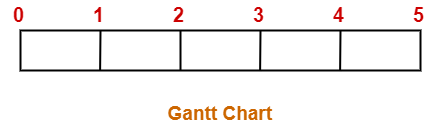Now,

• We take each job one by one in the order they appear in Step-01.
• We place the job on Gantt chart as far as possible from 0.

### Step-03:

• We take job J4.
• Since its deadline is 2, so we place it in the first empty cell before deadline 2 as-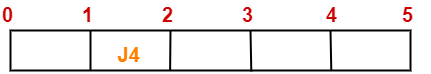### Step-04:

• We take job J1.
• Since its deadline is 5, so we place it in the first empty cell before deadline 5 as-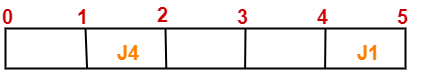### Step-05:

• We take job J3.
• Since its deadline is 3, so we place it in the first empty cell before deadline 3 as-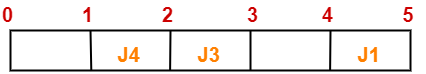### Step-06:

• We take job J2.
• Since its deadline is 3, so we place it in the first empty cell before deadline 3.
• Since the second and third cells are already filled, so we place job J2 in the first cell as-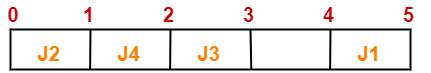### Step-07:

• Now, we take job J5.
• Since its deadline is 4, so we place it in the first empty cell before deadline 4 as-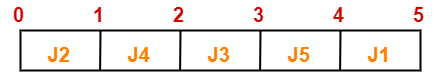Now,

• The only job left is job J6 whose deadline is 2.
• Thus, job J6 can not be completed.

Now, the given questions may be answered as-

### Part-01:

The optimal schedule is-

J2 , J4 , J3 , J5 , J1

This is the required order in which the jobs must be completed in order to obtain the maximum profit.

### Part-02:

• All the jobs are not completed in optimal schedule.
• This is because job J6 could not be completed within its deadline.

### Part-03:

Maximum earned profit

= Sum of profit of all the jobs in optimal schedule

= Profit of job J2 + Profit of job J4 + Profit of job J3 + Profit of job J5 + Profit of job J1

= 180 + 300 + 190 + 120 + 200

= 990 units

Watch this Video Lecture

Next Article- Huffman Coding

Get more notes and other study material of Design and Analysis of Algorithms.

Watch video lectures by visiting our YouTube channel LearnVidFun.

SummaryArticle Name
Job Sequencing With Deadlines | Algorithm | Example
Description
Job Sequencing with Deadlines- The sequencing of jobs on a single processor with deadline constraints is called as Job Sequencing with Deadlines. Greedy Algorithm is adopted to solve this problem. Job Sequencing with Deadlines Example.
Author
Publisher Name
Gate Vidyalay
Publisher Logo